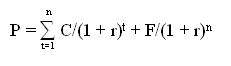# What is Yield to Maturity?

Yield to maturity is the rate of return expected on a bond if it is held until its maturity date. The concept is used by investors to evaluate the returns on different bond investments that may have a range of maturities and different coupon amounts. The rate of return is based on the stream of future coupon payments, the eventual return of principal, and the initial purchase price.

The specific inputs to the calculation are:

• Par value of the bond
• Market value of the bond
• Annual interest rate
• Maturity of the bond in years
• Timing of the coupon payments (such as quarterly, semi-annually, or annually)

If a bond holder sells the bond prior to its maturity date, the yield to maturity on that investment may vary from the amount that would have been realized if it had been held to the maturity date.

How it calculate

To understand YTM, one must first understand that the price of a bond is equal to the present value of its future cash flows, as shown in the following formula:Where:

P = price of the bond
n = number of periods
C = coupon payment
r = required rate of return on this investment
F = maturity value
t = time period when payment is to be received

Several issues included in the yield to maturity topic are:

• It discounts cash flows to their present value
• The concept is expressed as an annual rate of return
• All coupon payments are assumed to be reinvested at the same rate of return as the current yield to maturity
• All coupon payments are assumed to be paid on time
• There is no consideration of the transaction costs required to buy or sell a bond
• There is no consideration of the tax rate paid by the investor

The calculation of yield to maturity can be performed manually, but requires a series of trial and error estimates to gradually refine the result. A much easier approach is to plug the necessary information into a formula in an electronic spreadsheet.